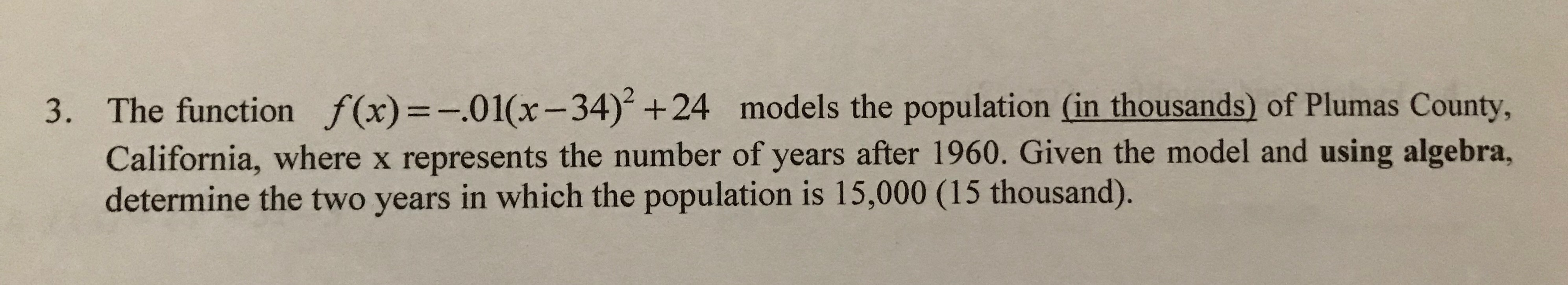# The function f(x)=-.01(x-34) +24 models the population (in thousands) of Plumas County, 3. California, where x represents the number of years after 1960. Given the model and using algebra, determine the two years in which the population is 15,000 (15 thousand).

Questionhelp_outlineImage TranscriptioncloseThe function f(x)=-.01(x-34) +24 models the population (in thousands) of Plumas County, 3. California, where x represents the number of years after 1960. Given the model and using algebra, determine the two years in which the population is 15,000 (15 thousand). fullscreen

### Want to see this answer and more?

Experts are waiting 24/7 to provide step-by-step solutions in as fast as 30 minutes!*